# Difference between revisions of "Space of path components"

## Definition

### As a set and pointed set

Suppose$X$ is a topological space (we sometimes take a based topological space, but the basepoint turns out to be irrelevant). The set of path components of$X$, denoted$\pi_0(X)$, is defined as follows:

• As a set, it is the set of path components of$X$. In other words, it is the set of equivalence classes of$X$ under the equivalence relation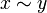$x \sim y$ iff there is a path from$x$ to$y$ in$X$.
• If$X$ is nonempty and$x \in X$, then$\pi_0(X,x)$, as a pointed set, is defined as the set$\pi_0(X)$ with the chosen basepoint being the element of$\pi_0(X)$ that is the path component of$x$.

### As a topological space

As a topological space, the space of path components is obtained by taking the quotient topology of$X$ under the equivalence relation$x \sim y$ iff there is a path from$x$ to$y$ in$X$.

For a locally path-connected space, the space of path components is a discrete space. Thus, often, when dealing with such spaces, we ignore the topology.

### As a monoid

If$X$ is a topological group, topological monoid, or (most generally) a H-space, then$\pi_0(X)$ naturally acquires the structure of a monoid. The structure is obtained by quotienting the original monoid (or H-space multiplication) by the equivalence relation of being path-connected.

In order to perform this quotienting, we need to justify that the relation of being in the same path component is a congruence for the original monoid (or H-space multiplication). This is indeed guaranteed by the continuity of multiplication: if$x_1$ and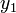$y_1$ are in the same path component, and$x_2$ and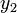$y_2$ are in the same path component, then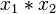$x_1 * x_2$ and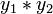$y_1 * y_2$ are in the same path component, because the paths can be multiplied pointwise.

Further, even if the original multiplication was not associative but only associative up to homotopy (making it an H-space), the new multiplication is strictly associative because any homotopy must descend to the identity map at the level of path components.

However, the multiplication need not be continuous on$\pi_0(X)$. Therefore,$\pi_0(X)$ nee not acquire the structure of a topological monoid.